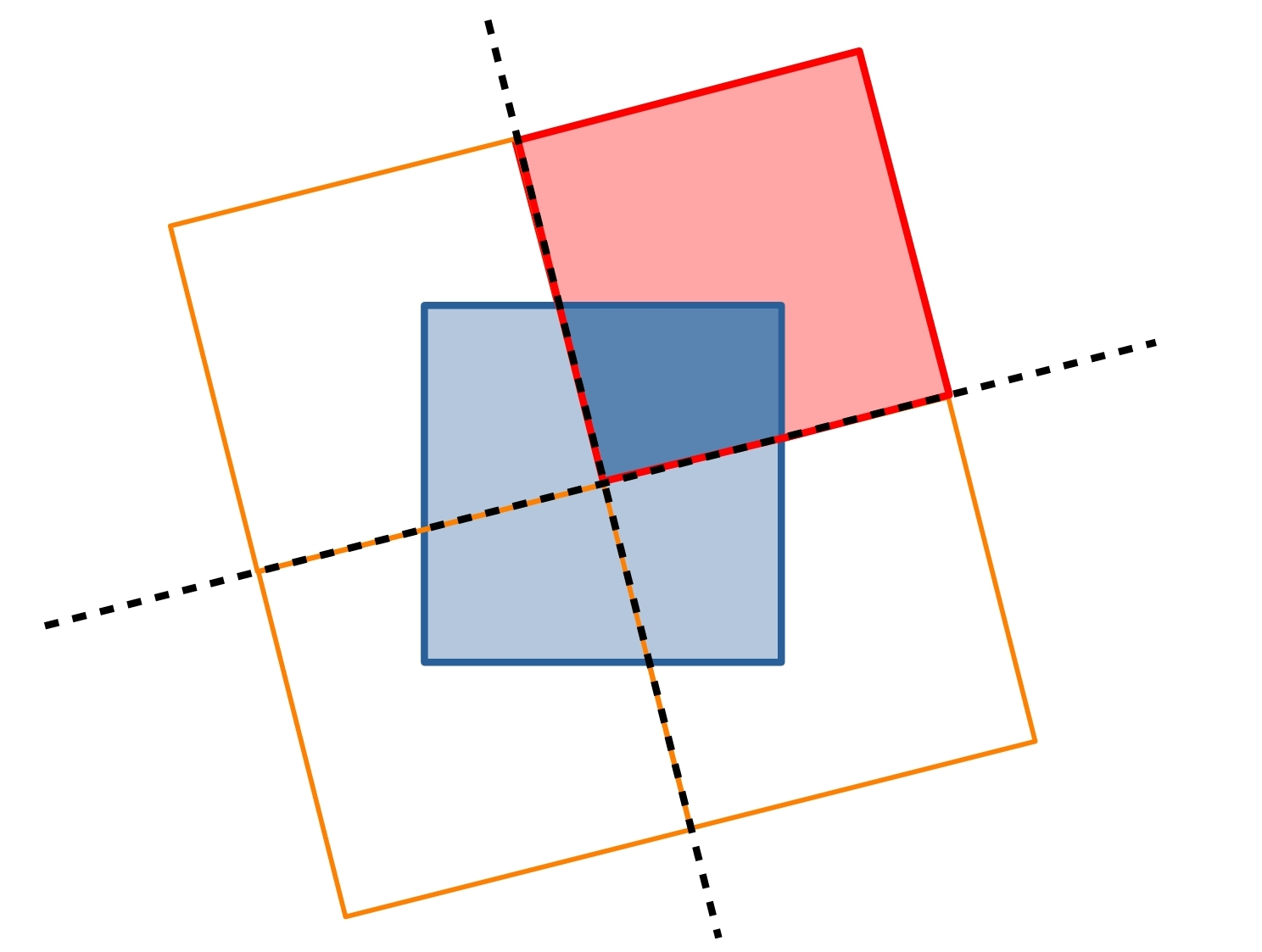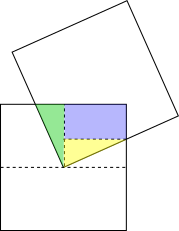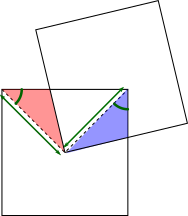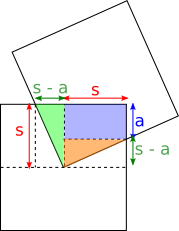#### You may also like### At a Glance

The area of a regular pentagon looks about twice as a big as the pentangle star drawn within it. Is it?### Six Discs

Six circular discs are packed in different-shaped boxes so that the discs touch their neighbours and the sides of the box. Can you put the boxes in order according to the areas of their bases?### Equilateral Areas

ABC and DEF are equilateral triangles of side 3 and 4 respectively. Construct an equilateral triangle whose area is the sum of the area of ABC and DEF.

# Overlap

##### Age 14 to 16Challenge Level

A red square and a blue square of the same size are overlapping so that the corner of the red square rests on the centre of the blue square.  Try using the slider to see how the overlap changes as the red square rotates.

Can you show that, whatever the orientation of the red square, it covers a quarter of the blue square?

Here are some ideas that you can use to help you do this:This picture shows what happens if you rotate the red square by $90^{\circ}$, $180^{\circ}$ and $270^{\circ}$ around the centre of the blue square.Can you use congurent triangles to complete this proof?Can you use congruent triangles again to find a different proof?In this method, let each side of the blue square have length $2s$.

Can you use this diagram, and the areas of rectangles and triangles, to find a fourth proof?

If the red square is smaller than the blue square what is the smallest length its side can have for your proof to remain true?

We are very grateful to the Heilbronn Institute for Mathematical Research for their generous support for the development of this resource.